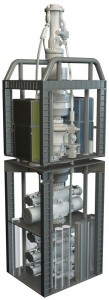# Accumulator Capacity – Usable Volume per Bottle Calculation for Subsea BOP

For subsea applications, hydrostatic pressure exerted by the hydraulic fluid must be accounted for calculation.In this case, we assume water depth at 1500 ft, therefore hydrostatic pressure exerted by hydraulic fluid (hydraulic fluid pressure gradient = 0.445 psi/ft) = 0.445×1500 = 668 psi. Besides of that, the concept for calculation is as same as surface accumulator. So please take a look about how to calculate usable volume per bottle as following steps.

Step 1 Adjust all pressures for the hydrostatic pressure of the hydraulic fluid:

Pre-charge pressure = 1000 psi + 668 psi = 1668 psi

Minimum system pressure = 1200 psi + 668 psi = 1868 psi

Operating pressure = 3000 psi + 668 psi = 3668 psi

Step 2 Determine hydraulic fluid required to increase pressure from pre-charge pressure to minimum system pressure:

Boyle’s Law for ideal gase: P1 V1 = P2 V2

1668 psi x 10 = 1868 x V2

16,680 ÷1,868 = V2

V2 = 8.93 gal

It means that N2 will be compressed from 10 gal to 8.93 gal in order to reach minimum operating pressure. Therefore, 1.07 gal (10.0 – 8.93 = 1.07 gal) of hydraulic fluid is used for compressing to minimum system pressure.

Step 3 Determine hydraulic required increasing pressure from pre-charge to operating pressure:

P1 V1 = P2 V2

1668 psi x 10 gal = 3668 psi x V2

16,680 ÷ 3668 = V2

V2 = 4.55 gal

It means that N2 will be compressed from 10 gal to 4.55 gal in order to reach operating pressure. Therefore, 5.45 gal (10.0 – 4.55 = 5.45 gal) of hydraulic fluid is used for compressing to operating pressure.

Step 4 Determine usable fluid volume per bottle:

Usable volume per bottle = Total hydraulic fluid/bottle – Dead hydraulic fluid/bottle

Usable volume per bottle = 5.45 – 1.07

Usable volume per bottle = 4.38 gallons

Share the joyWorking in the oil field and loving to share knowledge.

### 2 Responses to Accumulator Capacity – Usable Volume per Bottle Calculation for Subsea BOP

1.fein blade says:

2.Balenciaga outlet says: